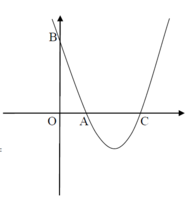# Finding perimeter and area using integration

#### Philip K.

##### New member
Hello,
I have the following problem:
Given the parabola y=x^2-4x+3 find the area and the parimeter of OAB
A=(1,0), B=(0,3), C=(3,0)

I calculated the area

$$\displaystyle S oab= \int ^{1}_{0}\left( x^{2}-4x+3\right) dx =\left| \left( \dfrac {x^{3}}{3}-\dfrac {4x^{2}}{2}+3x\right) \right| \ = \left| \left( \dfrac {1}{3}-\dfrac {4}{3}+3\right) -0\right| \ = \dfrac {4}{3}$$

However i am stuck into the parimeter.

\displaystyle P oab=\left| OA\right| +\left| OB\right| +\left| AB\right|\\ = 1 +3 + \int ^{1}_{0}\sqrt {1+\left( \dfrac {d}{dy}\right) ^{2}dx}\\ \begin{aligned}=4+\int ^{1}_{0}\sqrt {1+\left( 2x-4\right) ^{2}}d_{x}\\ =4+\int ^{1}_{0}\sqrt {4x-16x+12}dx\\ =4+2\int ^{1}_{0}\sqrt {x^{2}-4x+\dfrac {17}{4}}dx\\ =4+2\int ^{1}_{0}\sqrt {\left( x-2\right) ^{2}+\dfrac {1}{4}}dx\end{aligned}

Here i tried to substitute u= x-2, but i couldnt solve it.

#### HallsofIvy

##### Elite Member
I don't understand this. You give the parabola y= x^2- 4x+ 3 but then you ask for the are and perimeter of OAB which is a right triangle (with area (1/2)( 1)(3)= 3/2 and perimeter $$\displaystyle 1+ 3+ \sqrt{1+ 9}= 4+ \sqrt{9}$$) but has nothing to do with the parabola. Further you give a point, C, that lies on the parabla but has nothing to do with the triangle! The area you calculated is the area between the x-axis and the parabola between x= 1 and x= 3 which, again, has nothing to do with the triangle!

#### Philip K.

##### New member
I don't understand this. You give the parabola y= x^2- 4x+ 3 but then you ask for the are and perimeter of OAB which is a right triangle (with area (1/2)( 1)(3)= 3/2 and perimeter $$\displaystyle 1+ 3+ \sqrt{1+ 9}= 4+ \sqrt{9}$$) but has nothing to do with the parabola. Further you give a point, C, that lies on the parabla but has nothing to do with the triangle! The area you calculated is the area between the x-axis and the parabola between x= 1 and x= 3 which, again, has nothing to do with the triangle!
Here is the graph.#### HallsofIvy

##### Elite Member
So you are NOT asked to find the "area and perimeter of OAB"! That was what was confusing me. You are asked find the area and perimeter of the region bounded by the x and y axes and the parabola.

Yes, the area is the integral $$\displaystyle \int_0^1 x^2- 4x+ 3 dx= \left[\frac{1}{3}x^3- 2x^2+ 3x\right]_0^1= \frac{1}{3}- 2+ 3= \frac{1- 6+ 9}{3}= \frac{4}{3}$$ as you say.

To do the integral for arclength, $$\displaystyle \int_0^1 \sqrt{(x- 2)^2+ \frac{1}{4}} dx$$, start by letting u= x- 2 and du= dx. When x= 0, u= -2 and when x= 1, u= -1 so the integral becomes $$\displaystyle \int_{-2}^{-1} \sqrt{u^2+ 1/4}du$$. Factor out that $$\displaystyle \frac{1}{4}$$: $$\displaystyle \frac{1}{2}\int_{-2}^{-1} \sqrt{4u^2+ 1}du$$.

Do recognize that? If not, recall the identity $$\displaystyle sin^2(\theta)+ cos^2(\theta)= 1\(\displaystyle and divide through by \(\displaystyle cos^2(\theta)$$ to get $$\displaystyle \frac{sin^2(\theta)}{cos^2(\theta)}+ 1= \frac{1}{cs^2(\theta)}$$ or $$\displaystyle tan^2(\theta)+ 1= sec^2(\theta)$$. So, if se let $$\displaystyle 4u= tan(\theta)$$, $$\displaystyle \sqrt{4u^2+ 1}= \sqrt{tan^2(\theta)}= sec(\theta)$$. When u= -2, $$\displaystyle tan(\theta)= -8$$ so what is $$\displaystyle \theta$$. When u= -1. $$\displaystyle tan(\theta)= -4$$ so what is $$\displaystyle \theta$$?\)\)

#### Philip K.

##### New member
I dont get that "When x= 0, u= -2 and when x= 1, u= -1 "
The rest i understand

#### HallsofIvy

##### Elite Member
You had $$\displaystyle (x- 2)^2- \frac{1}{4}$$. I suggested letting u= x- 2 so that would become $$\displaystyle u^2-\frac{1}{4}$$. Your original integral was from x= 0 to x= 1. When x= 0, u= 0- 2= -2 and when x= 1, u= 1- 2= -1.

Last edited:
•Philip K.